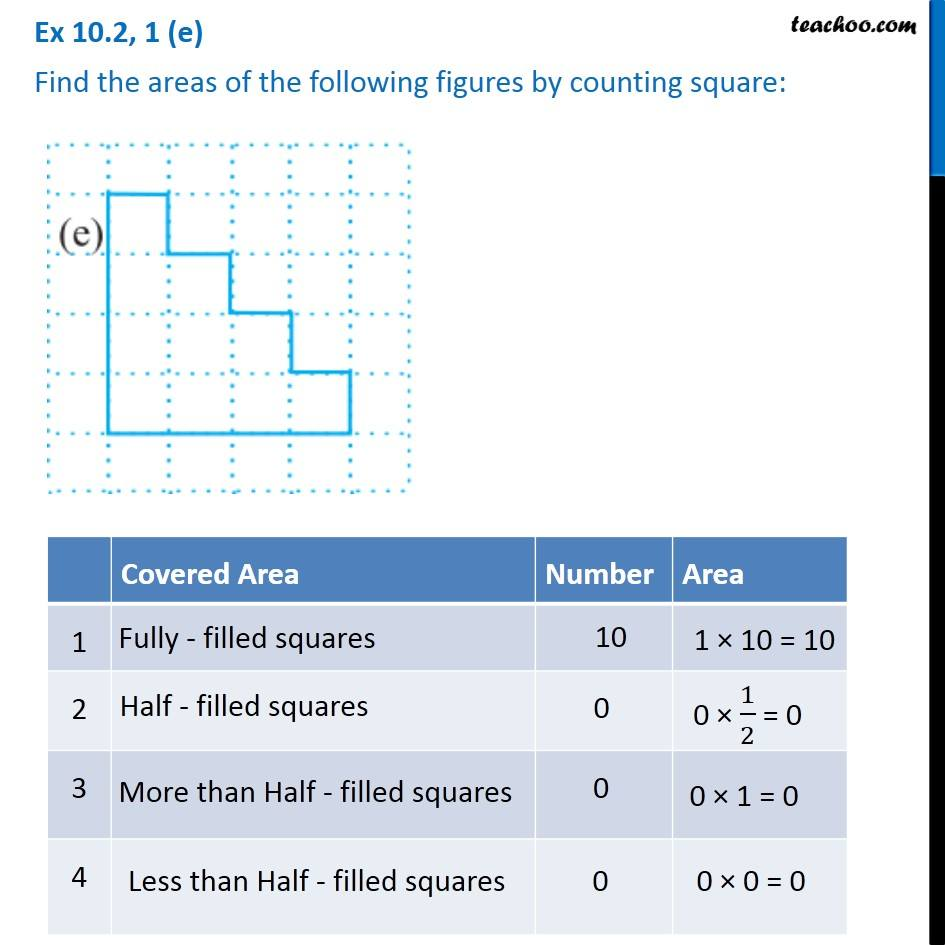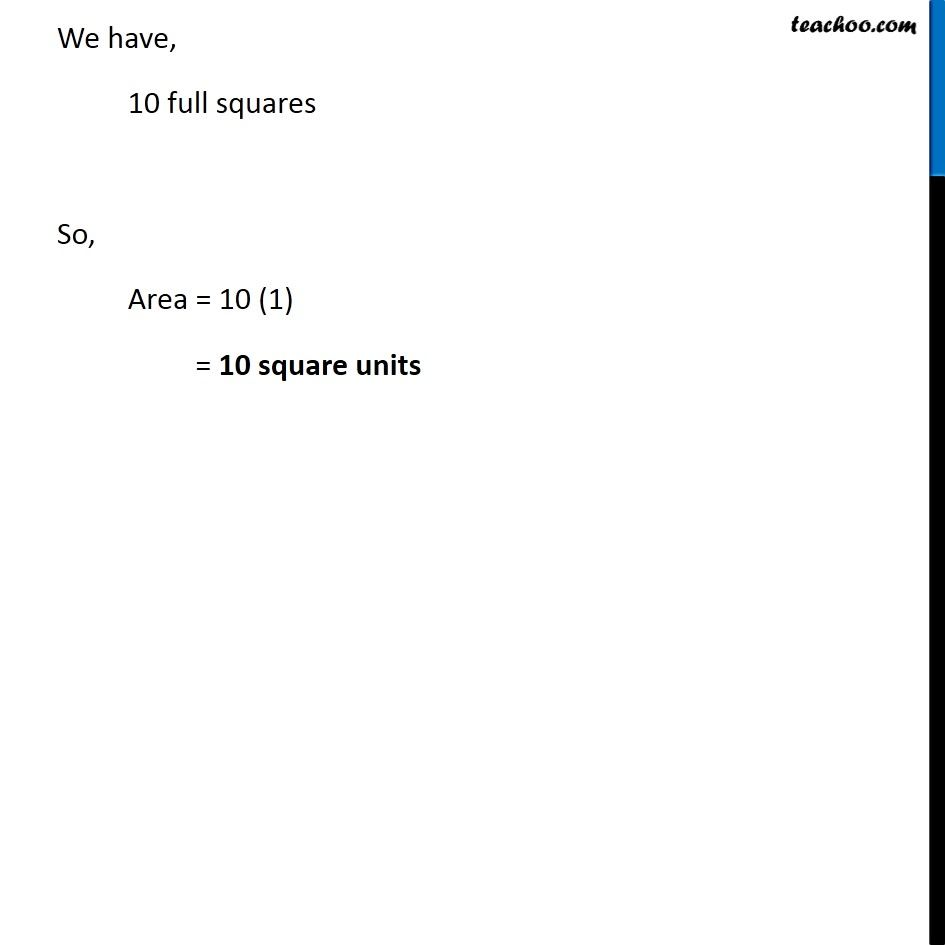Ex 10.2

Chapter 10 Class 6 Mensuration
Serial order wiseLearn in your speed, with individual attention - Teachoo Maths 1-on-1 Class

### Transcript

Ex 10.2, 1 (e) Find the areas of the following figures by counting square: Covered Area Number Area 1 Fully filled squares 10 1 × 10 = 10 2 Half filled squares 0 0 × 1/2 = 0 3 More than Half filled squares 0 0 × 1 = 0 4 Less than half filled squares 0 0 × 0 = 0 We have, 10 full squares So, Area = 10 (1) = 10 square units